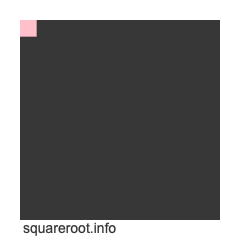Square Inches to Square Feet ConverterWelcome to the Square Inches to Square Feet Converter, also known as the sqin to sqft converter, in2 to ft2 converter, and in² to ft² converter.

To create a formula to convert square inches to square feet, we start with the fact that one inch equals one twelfth of a foot. Therefore, this formula is true:

Inches × (1/12) = Feet

However, we are dealing with square inches and square feet, which means inches and feet to the 2nd power. Thus, we take both sides of the formula above to the 2nd power to get this result:

Inches² × (1/12)² = Feet²
Inches² × (1/144) = Feet²
in² × (1/144) = ft²

Below is an illustration showing you how one square inch fits into one square foot. The cut out in pink in the top left corner is one square inch. You can fit 144 square inches into one square foot.Without further ado, please enter your square feet into the converter below so we can use our formula created above to convert it to square feet for you.

Convert  square inches to square feet.

Below are some examples of conversions that our Square Inches to Square Feet Converter can solve for you.

1 square inch to square feet

1.5 square inches to square feet

10 square inches to square feet

12 square inches to square feet

50 square inches to square feet

200 square inches to square feet

1000 square inches to square feet